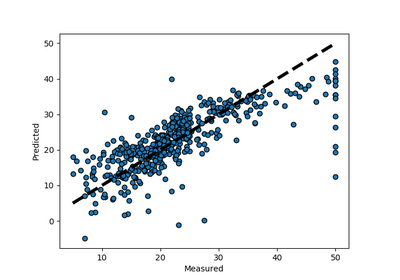# `sklearn.model_selection`.cross_val_predict¶

`sklearn.model_selection.``cross_val_predict`(estimator, X, y=None, groups=None, cv=None, n_jobs=1, verbose=0, fit_params=None, pre_dispatch=‘2*n_jobs’, method=’predict’)[source]

Generate cross-validated estimates for each input data point

Read more in the User Guide.

Parameters: estimator : estimator object implementing ‘fit’ and ‘predict’ The object to use to fit the data. X : array-like The data to fit. Can be, for example a list, or an array at least 2d. y : array-like, optional, default: None The target variable to try to predict in the case of supervised learning. groups : array-like, with shape (n_samples,), optional Group labels for the samples used while splitting the dataset into train/test set. cv : int, cross-validation generator or an iterable, optional Determines the cross-validation splitting strategy. Possible inputs for cv are: None, to use the default 3-fold cross validation, integer, to specify the number of folds in a (Stratified)KFold, An object to be used as a cross-validation generator. An iterable yielding train, test splits. For integer/None inputs, if the estimator is a classifier and `y` is either binary or multiclass, `StratifiedKFold` is used. In all other cases, `KFold` is used. Refer User Guide for the various cross-validation strategies that can be used here. n_jobs : integer, optional The number of CPUs to use to do the computation. -1 means ‘all CPUs’. verbose : integer, optional The verbosity level. fit_params : dict, optional Parameters to pass to the fit method of the estimator. pre_dispatch : int, or string, optional Controls the number of jobs that get dispatched during parallel execution. Reducing this number can be useful to avoid an explosion of memory consumption when more jobs get dispatched than CPUs can process. This parameter can be: None, in which case all the jobs are immediately created and spawned. Use this for lightweight and fast-running jobs, to avoid delays due to on-demand spawning of the jobs An int, giving the exact number of total jobs that are spawned A string, giving an expression as a function of n_jobs, as in ‘2*n_jobs’ method : string, optional, default: ‘predict’ Invokes the passed method name of the passed estimator. For method=’predict_proba’, the columns correspond to the classes in sorted order. predictions : ndarray This is the result of calling `method`

Notes

In the case that one or more classes are absent in a training portion, a default score needs to be assigned to all instances for that class if `method` produces columns per class, as in {‘decision_function’, ‘predict_proba’, ‘predict_log_proba’}. For `predict_proba` this value is 0. In order to ensure finite output, we approximate negative infinity by the minimum finite float value for the dtype in other cases.

Examples

```>>> from sklearn import datasets, linear_model
>>> from sklearn.model_selection import cross_val_predict
## Examples using `sklearn.model_selection.cross_val_predict`¶